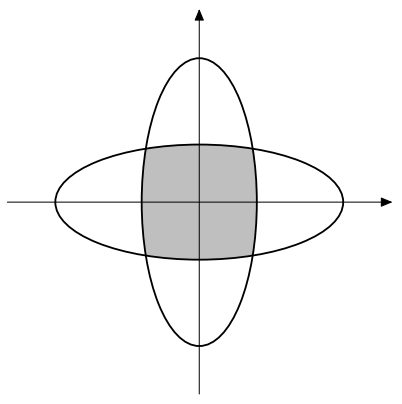시간 제한 메모리 제한 제출 정답 맞은 사람 정답 비율
8 초 (추가 시간 없음) 512 MB 0 0 0 0.000%

## 문제

Recently the astronomers have discovered a peculiar pair of planets, named A and B. As we know, a planet usually moves in an ellipse orbit, so do A and B. But their orbits are quite special:

1. Their orbits are in the same plane and share the same center;
2. The segments formed by joining their own focuses respectively are perpendicular to each other.

If we denote the center as point O, and the focuses of orbit A as F1 and F2, then we can build Cartesian coordinates, with point O being the origin, and the line through F1 and F2 being the x-axis.

Here is a sample:Figure 1: Sample orbits

As the astronomers would like to know more about the planets, they decide to calculate the intersection area first. But unfortunately calculating the intersection area is so complex and beyond their ability, so they have to turn to you, a talent of programming.

You are given the description of two ellipse orbits satisfying the conditions above, and required to calculate their intersection area.

## 입력

Input may contain multiple test cases. The first line is a positive integer n (n ≤ 100), denoting the number of test cases below. Each case is composed of two lines, the first one is the description of orbit A, and the second one the description of orbit B. Each description contains two positive integers, a and b (a, b ≤ 100), denoting the ellipse equation x2/a2 + y2/b2 = 1. It is guaranteed that the focuses of orbit A are on x-axis, and the focuses of orbit B are on y-axis.

## 출력

For each test case, output one line containing a single real number, the area of the intersection, with an error up to 0.001.

## 예제 입력 1

1
2 1
1 2


## 예제 출력 1

3.709